Sample Final Exam        MAT 131, Fall 1998

The final will be held on Thursday, December 17 at 7:00 pm. Be sure to bring your Stony Brook ID card and your calculator. You may also bring a single" by 11" sheet of handwritten notes. This sheet must not be a photocopy or computer printout. The locations of the final exam are given in the table below.

 1 Barcus/Perez Physics P113 2 Barcus/Perez Physics P118 3/4 Sutherland/Kim Humanities 101 5 Mandell/Herrera-Guzman SB Union 236 6 Mandell/Herrera-Guzman SB Union 237 7/8 Bernhard Earth+Space 001 9 Bishop/Panafidin Lt Engineer 152 10 Bishop/Panafidin Hvy Engineer 201 11/12 Barcus/Weinberg SB Union Aud. 13 Schalm/Cheng SB Union Aud. 14 Schalm/McKenzie SB Union Aud. 15 Schalm/Cheng SB Union Aud. 16 Schalm/Moraru SB Union Aud. 17/18 Bernhard/Teo Earth+Space 001

This sample is a collection of problems similar to the type of questions which will be on the final. However, you are responsible for all the material we have covered this semester-- just because a topic isn't on this sample doesn't mean it won't be on the final. Also, merely completing this sample exam is not adequate preparation for the final. You must also do a large number of additional problems, both those assigned in the homework and others like them. You should ensure that you know how to do all the problems on the midterms and the previous sample exams, as well. This sample contains more problems than the actual final.

1. Write the equation of the linear function f with f(0)=1 and f(3)=3. Also write the equation of the exponential function g with g(0)=1 and g(3)=3.

2. Compute the derivatives with respect to x for each of the following:

(a)
x-1/2 + x + x1/2
(b)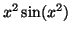(c)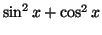(c)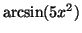(d)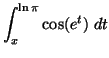(e)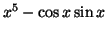(f)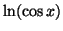(g)(h)3. Compute each of the following anti-derivatives (indefinite integrals):

(a)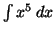(b)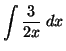(c)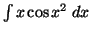(d)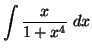(e)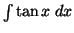(f)4. Evaluate each of the following definite integrals:

(a)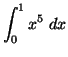(b)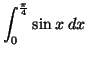(c)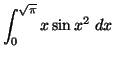(d)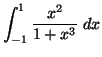(e)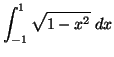(f)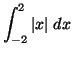5. What is the average value of y=x3 over the interval [0,2]?

6. Find the point on the graph of the curve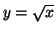that is closest to the point (5,0). (Hint: if d is the distance from (5,0) to a point on the curve, then it is permissible (and easier!) to minimize d2.)

7. Give the left-hand sum, right-hand sum, and trapezoid approximation for the integral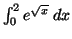, using n=4 rectangles. What should n be to ensure that the right-hand sum is accurate to within 0.001? (Hint: compare the expressions for the left-hand and right-hand sums for arbitrary n-- what does this tell you about the exact value of the integral?)

8. Write the equation of the line tangent to the curve y=3x2 + 2x + 1at the point (1,6).

9. Write the equation of the line tangent to the curve y3-2xy+x3=0at the point (1,1).

10. From physics, we know that the illumination at a point xwhich is provided by a light source at L is proportional to the intensity of the light at L divided by the square of the distance between x and L. Suppose that two lights L1 and L2 are placed 20 meters apart, and that the intensity of L2 is 8 times the intensity of L2. Where is the point on the line between L1 and L2 where the illumination is at a minimum?

11. Find the maximimum and minimum values of the function f(x)=x3 + 3x2 - 42x - 22 on the interval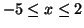.

12. Calculate the area of the region bounded by the graphs of y=x/2 and x=y2-3.

13. Write the equation of the parabola which best approximates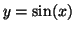at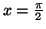(that is, the second Taylor polynomial). Use your polynomial to find approximations of the nonzero solutions to. (Hint: graph the relevant functions for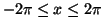to make sure your answers make sense. The fact that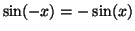is helpful.)

14. Use Newton's method to determine the nonzero solutions of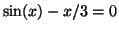to within 0.000005. You may either use your answer to the previous problem as x0, or use x0=2.

15. Compute the following limits. Distinguish between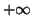,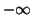, and does not exist''.

(a)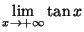(b)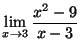(c)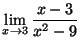(d)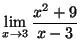(e)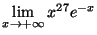(f)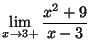16. The figure below is the graph of a function f(x). Use it to sketch the graph of f'(x) and the graph of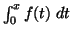.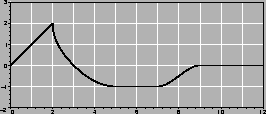17. For each differential equation on the left, indicate which function on the right is a solution.

 (a) y'=5y (1) y=x2 (b) y'=y(y-1) (2)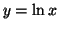(c) x2 y'' + 2x y' = 1 (3) y=(1+e-x)-1 (d) yy'' = xy' (4) y=e5x

18. A coffee filter has the shape of an inverted cone. Water drains from it at a constant rate of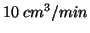. When the depth is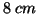, the water level drops at a rate of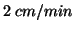. What is the ratio of the height of the cone to its radius? You may find it useful to recall that the volume of a cone of radius r and height h is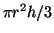.

19. Letbe the parametric curve given by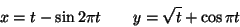What is the slope ofat the point (4,2), when t=4?

20. An angle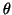is known to vary periodically with time, in such a way that its rate of change is proportional to the product of itself and the cosine of the time t. Write a differential equation which expresses this relationship. Show that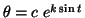is a solution to the differential equation. If you know that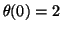and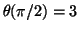, what is the equation for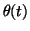?

Scott Sutherland
1998-12-07